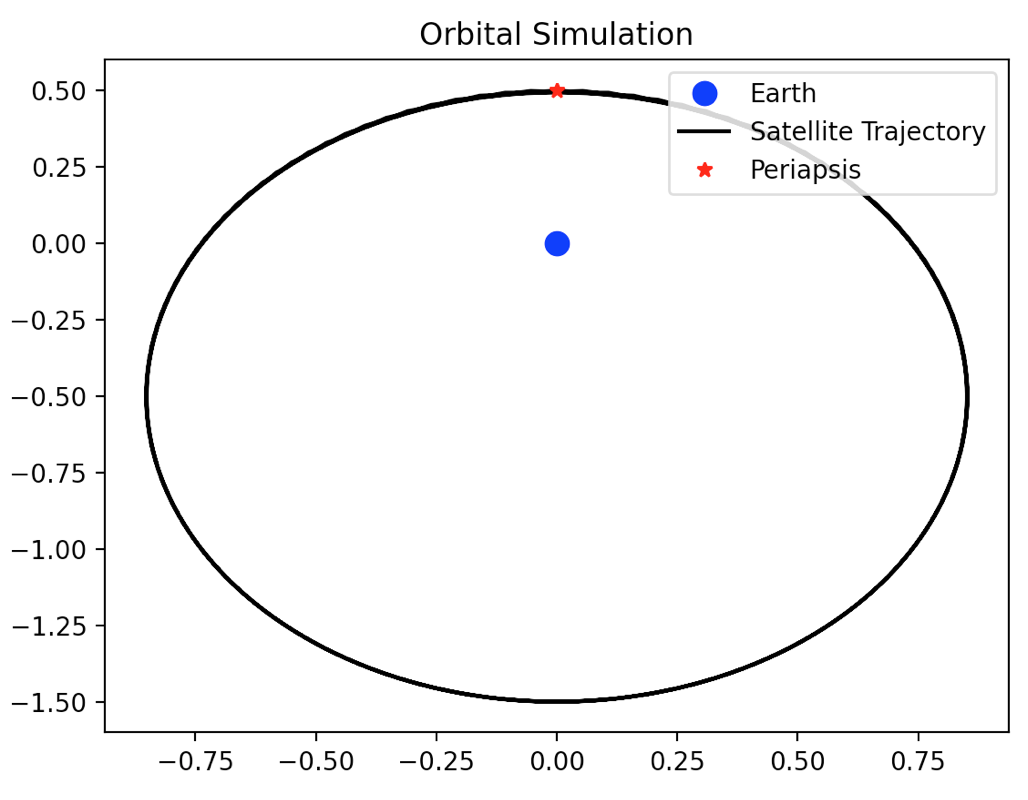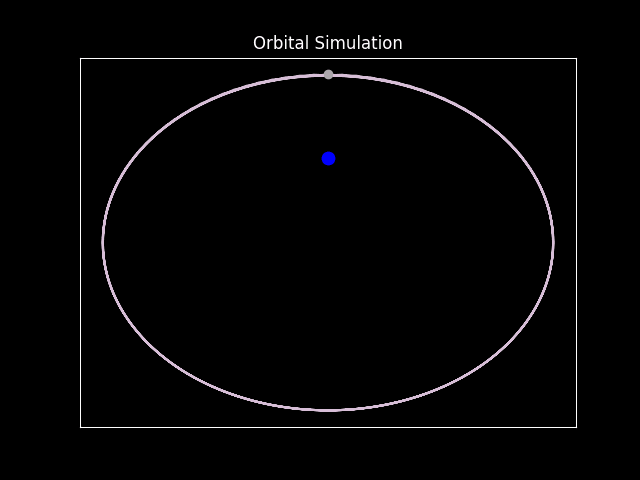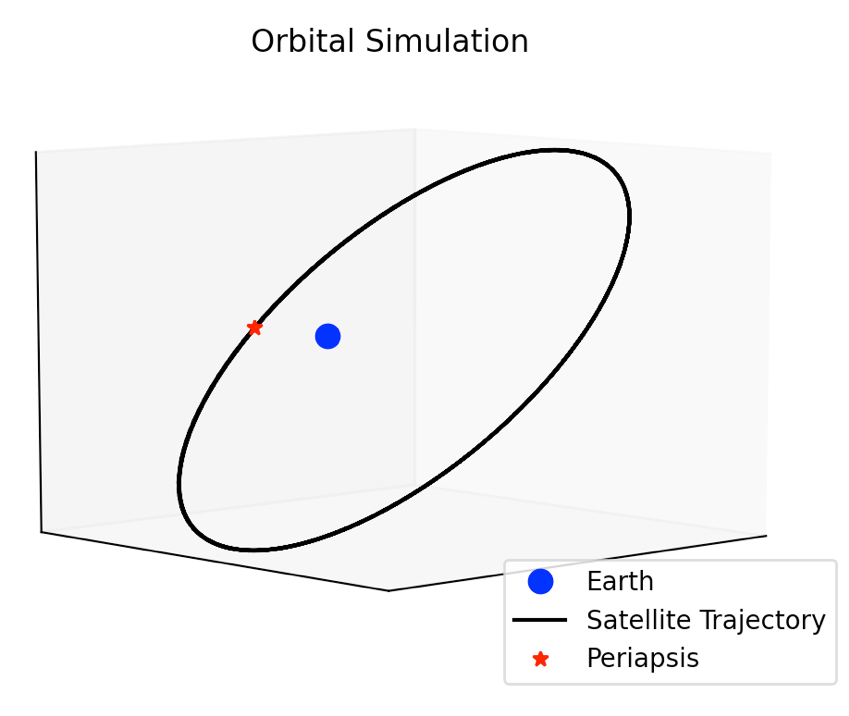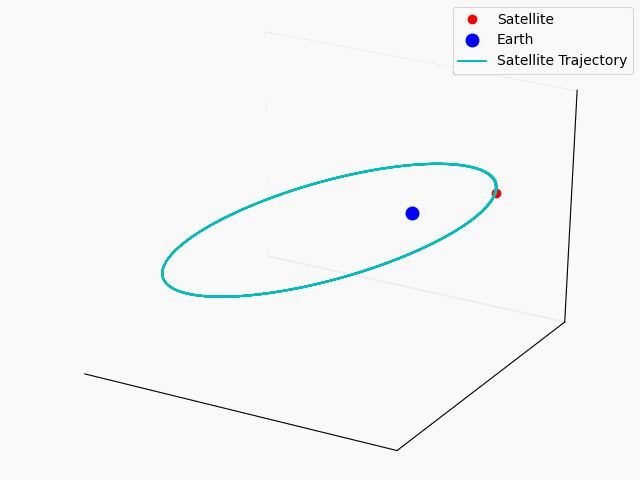This snippet represents approximately 1/3 of Chapter 5: Modeling a 2-body orbit in 2D and 3D. It is provided as a way for you to "test drive" the book.

# Chapter 5: Modeling a 2-body orbit in 2D and 3D

## Introduction

Python is a perfect candidate for modeling simple 2-body orbits like a satellite orbiting the Earth. (It’s also a good candidate for complicated 3-body orbits, but we won’t get into those in this book.) We want to be able to model basic circular and elliptical orbits in two dimensions and three dimensions. We’ll take advantage of Matplotlib’s `FuncAnimation()` function to animate the 2D orbit to show how the orbital velocity increases at the closest point to Earth and decreases at the farthest point from Earth. We can also use Matplotlib’s 3D plotting functionality to plot circular and elliptical orbits in 3D, which will help to illustrate the effects of the different orbital parameters.

Solving the 2-body problem requires some form of ordinary differential equations to solve for time and position. These methods usually require a type of numerical method (Newton’s Method, Runge-Kutta methods, Kepler’s Method, or lots of others) to numerically solve position as a function of time. We could write these differential equation solvers ourselves, but we can use existing libraries that have this functionality. We will use `PyAstronomy`’s `KeplerEllipse` class to create x, y, and z coordinates of a satellite’s position over a period of time, then we will use Matplotlib to plot these points. The documentation for PyAstronomy can be found at footnote  at the end of this chapter.

The particulars of the hows and whys of orbital mechanics are beyond the scope of this book; however, this paragraph will serve as a crash course in orbital mechanics. A satellite’s orbit around a body (we will use Earth in this chapter) can be described and calculated based on the following parameters:

• Semi-Major and Semi-Minor Axes (a): These are the largest and smallest radii measured from the body being orbited to the farthest and closest points in the orbit, respectively. Typically, only the semi-major axis is used as a parameter. For a circular orbit, the semi-major and semi-minor axes are equal, and we call it the radius.
• Eccentricity (e): This is a description of the shape of the orbit. An eccentricity of 0 means the orbit is perfectly circular; an eccentricity between 0 and 1 is an elliptical orbit. For example, an elliptical orbit with eccentricity = 0.50 means that the semi-major axis is twice as large as the semi-minor axis. An eccentricity of 1 is a parabolic orbit, which means that the satellite will slingshot around Earth once and will not return. An eccentricity greater than 1 is a hyperbolic orbit.
• Inclination (i): The angle between the Earth’s equator and the angle of the orbit. An orbit with 0 inclination stays over the equator; an orbit with 90 degrees inclination orbits over the North and South Poles. Typically, an orbit’s inclination coincides with the latitude at which it was launched: satellites launched from Cape Canaveral, FL at latitude 30 degrees North have an inclination of 30 degrees.
• Periapsis and Apoapsis: Periapsis is the closest point in the satellite’s orbit to the body being orbited, and apoapsis is the farthest point in the satellite’s orbit to the body being orbited. -apsis is the suffix for a general body, so every orbit has a periapsis. -gee is the suffix for Earth, so all satellites orbiting the Earth have a perigee. -helion is the suffix for the Sun, so all satellites orbiting the Sun have a perihelion.
• Argument of Periapsis (w): The angle between the point on the orbit that intersects the extended equator line and the closest point in the orbit to Earth (periapsis). A circular orbit with 0 inclination will have an argument of perigee of 0 degrees. This parameter essentially moves the closest point in the orbit to Earth to a different point along the orbit.
• Longitude of the Ascending Node (omega): The angle between a longitudinal reference (something like the Sun) and the direction of the ascending node, the point when the “climbing” part of the orbit intersects with the extended equator. This parameter can be used to “rotate” the orbit around the Earth.

Don’t worry if you don’t totally understand all of the parameters. That’s what visuals are for. With orbital mechanics out of the way, let’s get to Python. We’ll start with displaying a 2D static plot of the orbit of a satellite around Earth, animating the 2D plot of the orbit, then finish with displaying a 3D plot of the orbit around Earth.

## Imports

```import numpy as np from PyAstronomy import pyasl import matplotlib.pyplot as plt import matplotlib.animation as animation ```

PyAstronomy is not part of the default Anaconda distribution, so you will have to download it with `pip install PyAstronomy`.

## Body

The beauty of using a third party library (that has been properly vetted) is that the library can handle the hard work, and we only need one line to create the elliptical orbit object `KeplerEllipse`. We’ll start with a basic ellipse: semi-major axis of 1.0 units, a time period of 1.0 units, an inclination of 30 degrees, and everything else 0. We’ll also use numpy to create time intervals to be used in determining position later.

```orbit = pyasl.KeplerEllipse(a=1.0, per=1.0, e=0.5, Omega=0.0, i=30.0, w=0.0) t = np.linspace(0, 4, 200) ```

The `linspace` function accepts a starting point, and ending point, and a number of samples that you want in between. In this case, it calculates 200 samples between 0 and 4 (inclusive). It means that we don’t have to calculate the actual step size; numpy will do that for us. All that is left to do is call the `xyzpos()` method, which accepts a time array argument and returns a 3 column array the same length as the input time array. It is important to note that the returned array is in the format Y coordinates, X coordinates, Z coordinates. For a 2D plot, we’ll ignore the Z coordinates, so our viewpoint is looking down at the North Pole of Earth from above Earth.

`pos = orbit.xyzPos(t)`

Congratulations, that’s all of the orbital mechanics that is required! The rest of the program will deal with matplotlib and setting the proper parameters. Because of the convention used within PyAstronomy, the first point in `pos` corresponds to perigee, the closest point to the Earth. We will plot perigee separately so we have our own reference point when we tweak the orbital parameters. The array-like notation that is contained within `pos` means that we will have to use a peculiar double colon `∷` to get all of the elements within that array for that coordinate system. Earth will be at (0, 0, 0) in this coordinate system, and we’ll plot it with a big marker size so that we can see it on the plot.

```plt.plot(0, 0, 'bo', markersize=9, label="Earth") plt.plot(pos[::, 1], pos[::, 0], 'k-', label="Satellite Trajectory") plt.plot(pos[0, 1], pos[0, 0], 'r*', label="Periapsis") ```

Since `pos`'s array coordinates are in the system (Y, X, Z), to get the X coordinates, we need to call the “first” (in reality, the second) element in the array. Add a legend and a title, and let’s show the plot:

```plt.legend(loc="upper right") plt.title('Orbital Simulation') plt.show() ```